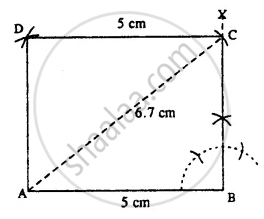# Construct a Rectangle Abcd; If : Ab = 5.0 Cm and Diagonal Ac = 6.7 Cm. - Mathematics

Sum

Construct a rectangle ABCD; if :

AB = 5.0 cm and diagonal AC = 6.7 cm.

#### SolutionSteps :

1. Draw AB = 5 cm.
2. At B, draw ∠XBA = 90°.
3. From A, draw an arc of radius 6.7 cm which meets XB at C.
4. From C, draw an arc of a radius of 5 cm.
5. From A, draw an arc of radius equal to BC which meets the first arc at D.
6. Join AD and CD. Thus ABCD is the required rectangle.
Concept: Construction of a Rectangle When Its Length and Breadth Are Given.
Is there an error in this question or solution?

#### APPEARS IN

Selina Concise Mathematics Class 8 ICSE
Chapter 18 Constructions
Exercise 18 (D) | Q 3.3 | Page 211